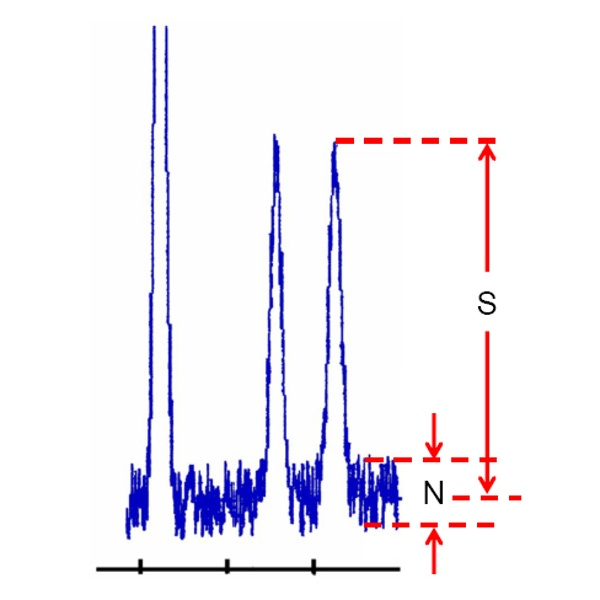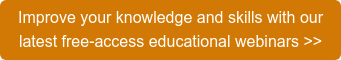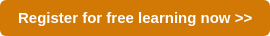# Liquid Chromatography

In HPLC Solutions #122, we looked at how to measure baseline noise from a liquid chromatogram. One practical application of such measurements is to determine the signal-to-noise ratio (S/N), which we’ll consider here.

Signal-to-noise often is used to help determine the limit of detection or limit of quantification of an HPLC method. To make this measurement, you’ll need to have a chromatogram under the desired conditions that contains enough baseline to measure the noise accurately – often 3-20 times the width of the peak. An example is shown in Figure 1.Figure 1

Expand the chromatogram sufficiently to facilitate the measurements, and transfer it to a graphics program or print a copy if it makes the measurements easier. First, measure the baseline noise, using the technique discussed in HPLC Solutions #122 to capture the width of the baseline, which is the noise, N, in Figure 1. Next, measure the signal, S, from the middle of the baseline noise vertically to the top of the peak of interest. My measurements are N = 66 and S = 367 (arbitrary units).

Most workers calculate S/N just as the ratio of the signal to the noise, so S/N = 367/66 = 5.56 in this example. However, The United States Pharmacopoeia (USP) and European Pharmacopoeia (EP) use a different calculation where only half of the noise band is considered. In their documentation, they call the signal H and the noise h, defining S/N = 2H/h. You can see that this reported value would give S/N = (2 x 367) / 66 = 11.1 in the present case, which is twice the intuitively defined ratio. In my opinion, this confusion in definitions is unfortunate, and is one reason why S/N may not be the best way to determine the lower limits of a method. We’ll consider limit tests in future discussions.

This blog article series is produced in collaboration with John Dolan, best known as one of the world’s foremost HPLC troubleshooting authorities. He is also known for his research with Lloyd Snyder, which resulted in more than 100 technical publications and three books. If you have any questions about this article send them to TechTips@sepscience.com### Posts by Topic